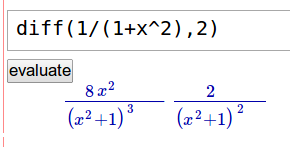# Revision history [back]

### Latex rending problem in notebook

Hello,

I'm using sage 5.5 under Ubuntu 12.04 with Chrome 24.0.1312.56.

Doing diff(1/(1+x^2),2) the minus sign does not display. On the command line the latex is:

sage: diff(1/(1+x^2),2) 8*x^2/(x^2 + 1)^3 - 2/(x^2 + 1)^2

sage: latex(diff(1/(1+x^2),2)) \frac{8 \, x^{2}}{{\left(x^{2} + 1\right)}^{3}} - \frac{2}{{\left(x^{2} + 1\right)}^{2}}

In the notebook, I see:Anything I can do to fix the rending?